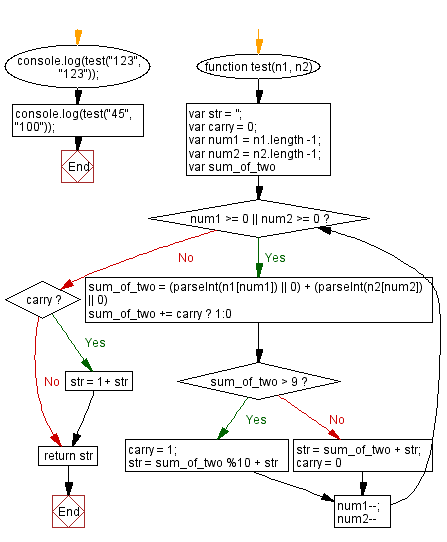# JavaScript: Two positive integers as string, return their sum

## JavaScript Math: Exercise-77 with Solution

Write a JavaScript program to compute the sum of two non-negative integers num1 and num2 represented by strings.

Test Data:
("123", "123") -> 246
("45", "100") -> 145

Sample Solution:

HTML Code:

``````<!DOCTYPE html>
<html>
<meta charset="utf-8">
<title>JavaScript program to Two positive integers as string, return their sum</title>
<body>

</body>
</html>
```
```

JavaScript Code:

``````function test(n1, n2) {
var str = '';
var carry = 0;
var num1 = n1.length -1;
var num2 = n2.length -1;
var sum_of_two

while(num1 >= 0 || num2 >= 0){
sum_of_two = (parseInt(n1[num1]) || 0) + (parseInt(n2[num2]) || 0)
sum_of_two += carry ? 1:0

if(sum_of_two > 9){
carry = 1;
str = sum_of_two %10 + str
}else{
str = sum_of_two  + str;
carry = 0
}
num1--;
num2--
}
if(carry){str = 1+ str}
return str
};
console.log(test("123", "123"));
console.log(test("45", "100"));
```
```

Sample Output:

```246
145
```

Flowchart:Live Demo:

See the Pen javascript-math-exercise-77 by w3resource (@w3resource) on CodePen.

Improve this sample solution and post your code through Disqus

What is the difficulty level of this exercise?

Test your Programming skills with w3resource's quiz.

﻿